# Java Program to Check if An Array Contains a Given Value

In this tutorial you will learn about the Java Program to Check if An Array Contains a Given Value and its application with practical example.

In this tutorial, we will learn to create a Java program to Check if An Array Contains a Given Value using Java programming.

## Prerequisites

Before starting with this tutorial we assume that you are best aware of the following Java programming topics:

• Java Operators.
• Basic Input and Output
• Class and Object.
• Basic Java programming.
• if-else statements.
• Nested if-else Statements.
• Java Scanner class.

## What is array?

An array is  a derived Data type that holds a fixed number of values of a single Type. An Array is a collection variable elements  that can store multiple values in a single variable of same type.In Computer Science,an array is a data structure, or simply an array, is a data structure consisting of a collection of elements.

## Java Program to Check if An Array Contains a Given Value

In this our program we will create a program to Check if An Array Contains a Given Value . We would first declared and initialized the required variables. Next, we would prompt user to input values . Later in program we will to Check if An Array Contains a Given Value.

## Output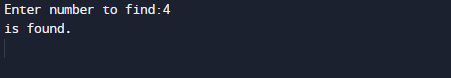In the above program, we have first declared and initialized a set variables required in the program.

• arr= it will hold array elements.
• find= it will hold given value form user.
• found= it will give us value is found or not.
• i = for iteration.

And  in the next statement user will be prompted to enter two values and  which will be assigned to variable ‘find‘ .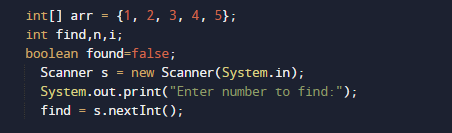Now, here we use  for loop to iterate through all elements of arr[]  and check if  we find is equal to any element in array or not.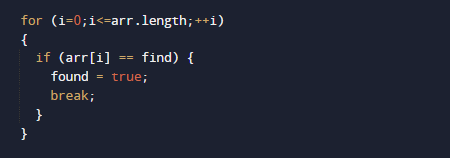If value is find , we set the value of found variable to true and break the loop.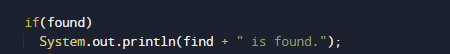If not, we move on to the next values in loop. at the end we  print found the value or not .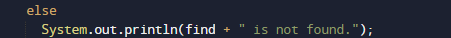In this tutorial we have learn about the Java Program to Check if An Array Contains a Given Value and its application with practical example. I hope you will like this tutorial.